• ## R语言：数据地图

千次阅读 多人点赞 2020-02-28 14:51:15
R语言：绘制新冠肺炎数据地图世界地图的绘制中国地图的绘制方法一：方法二： 数据地图是一种经典的图示方法，因此 世界地图的绘制 R包中存储着常见地图数据，比如maps包中包含世界地图、美国地图等 library(maps) ...
R语言：数据地图基本地图的绘制世界地图中国地图实例一：NBA球队实例二：新冠肺炎
数据地图是一种经典的图示方法，在R软件中，各种程序包所提供的函数在绘制数据地图时比较方便。
基本地图的绘制
世界地图
方法一
R包中存储着常见地图的数据，比如maps包中包含世界地图、美国地图等
library(maps)
map("world", fill = TRUE, col = rainbow(200),
ylim = c(-60, 90), mar = c(0, 0, 0, 0))
title("世界地图")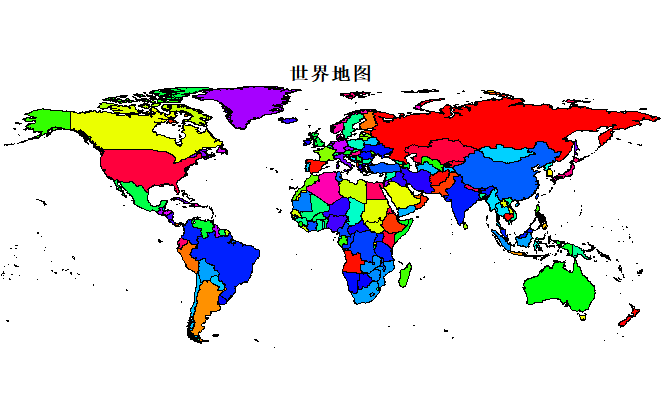方法二
library(maps)
data("world.cities")
bigcities <- subset(world.cities, pop > 5000000)
qplot(long, lat, data = bigcities,colour=country.etc,size=pop)+ borders("world", size= 0.5)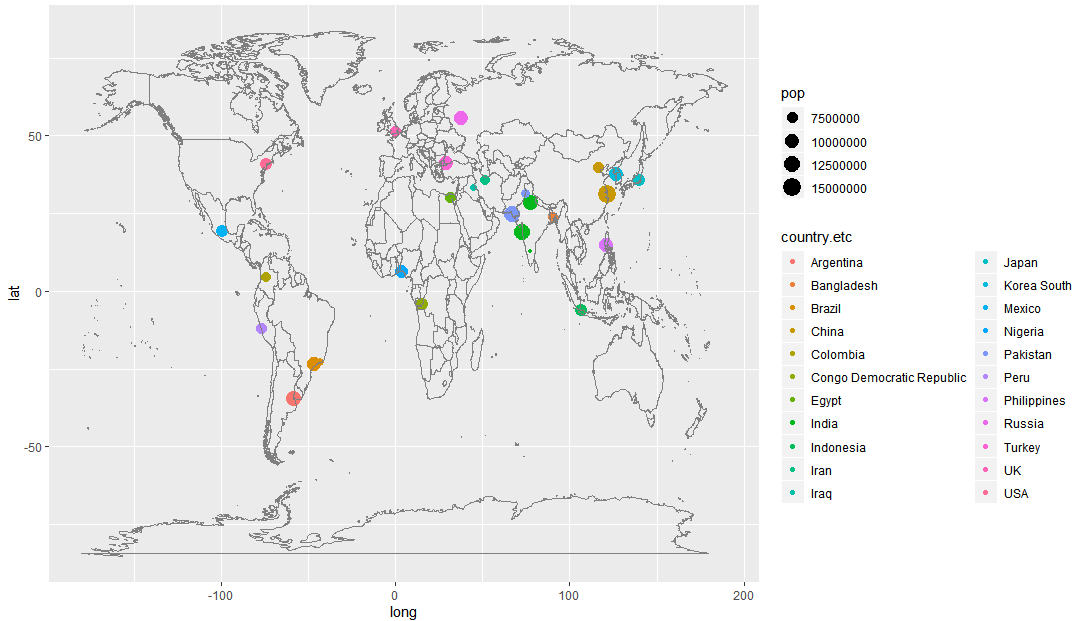中国地图
利用ggplot2绘制美国地图比较容易，因为美国地图是程序包maps中自带的地图，但其他国家的地图数据则需要从外部导入。
方法一
首先，安装相应的包
install.packages("mapproj")
install.packages(“sp”)
install.packages(“maptools”)
library(maptools)
library(ggplot2)
library(plyr)
library(sp)

下载中国地图的GIS数据
这是一个压缩包，完全解压后一般包含三个文件（bou2_4p.dbf、bou2_4p.shp和bou2_4p.shx），将这三个文件解压到R的工作空间下，或者直接输入路径：
x <- readShapePoly("C:/Users/SAMSUNG/Desktop/china-province-border-data/bou2_4p.shp")
plot(x)

library(rgdal)
china_map1 <- fortify(china_map)

#绘制全国地图-不着色
ggplot(china_map1,aes(x=long,y=lat,group=group))+
geom_polygon(fill="white",colour="black")+
coord_map("polyconic")

#绘制全国地图-分省着色
xs<-data.frame(x,id=seq(0:924)-1)
china_map_data<-join(china_map1, xs, type = "full")

ggplot(china_map_data, aes(x = long, y = lat))+
geom_polygon(aes(group = group,fill=NAME),color="grey40" )+ #线条色
coord_map("polyconic")+
scale_fill_manual(values=colours(),guide=FALSE) #分省着色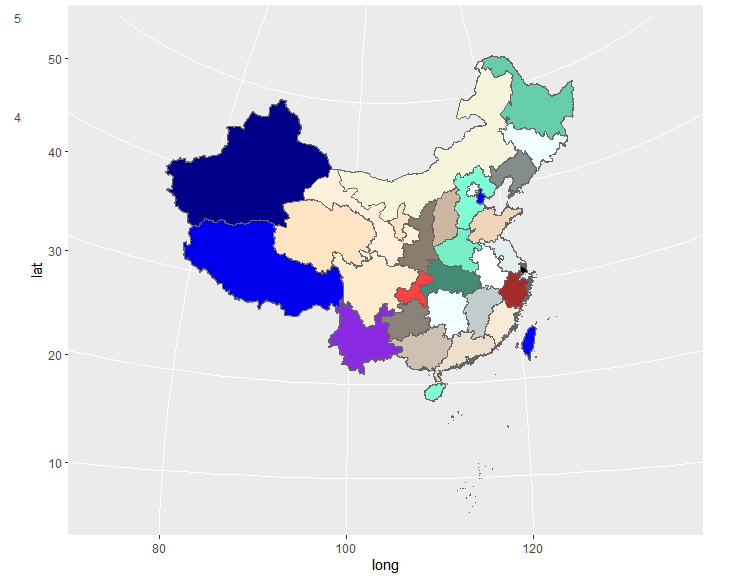方法二
但是。。。你会发现一些令人气愤的事，如下图【笔者已经无fuck说了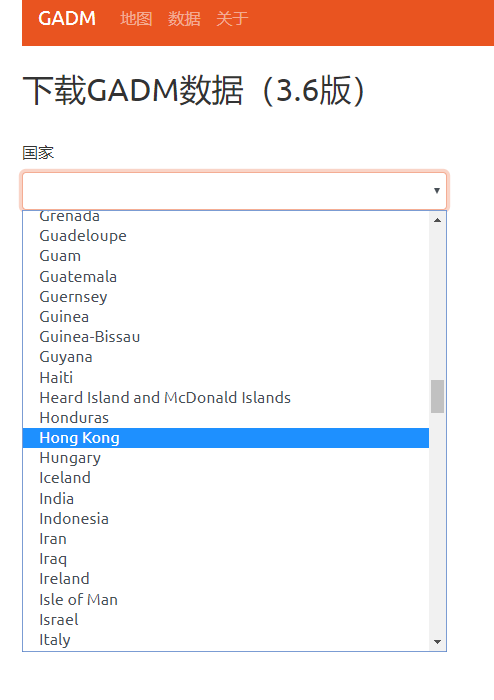代码如下，可自己补全路径
install.packages(“sp”)
library(sp)
gadm$pop=c(1961,1293,7185,3571,2470,4374,2745,3831,2301,7866,5442, 5950,3689,4456,9579,9402,5723,6570,10432,4602,867,2884, 8041,3474,4596,300,3732,2557,562,630,2181,706) #利用空间绘图命令进行绘图 spplot(gadm,"pop",col.regions = rev(terrain.colors(gadm$pop)),main="中国各省人口数据")

方法三
library(mapdata)
ch_cities <- subset(world.cities, country.etc=="China")
ggplot(ch_cities, aes(long, lat)) + geom_point(colour= alpha("red",0.5))+ borders("china")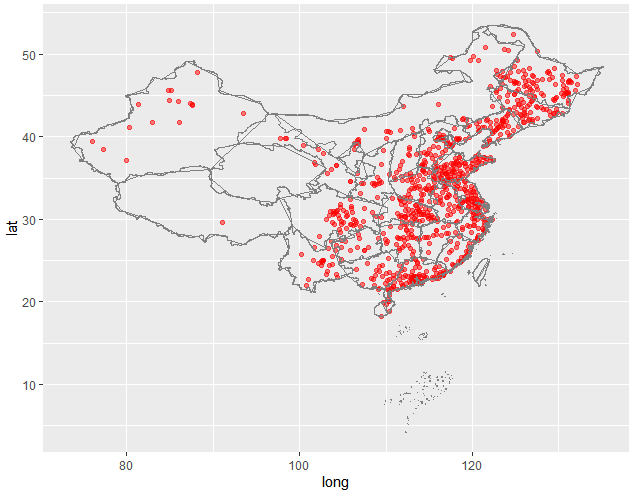方法四
map("china", col = "red4", ylim = c(18, 54), panel.first = grid())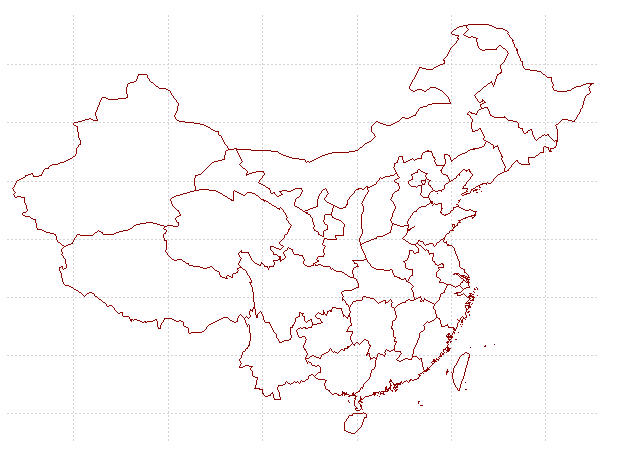实例一：NBA球队
NBA比赛球队30支,现想了解詹姆斯（LBJ）对阵哪个球队时表现更好，或得分更高，故通过绘制数据地图直观地得到结论。
library(ggplot2)

attach(lbj)
state_map=map_data("state")  #获取美国地图的数据信息

p=ggplot(lbj,aes(map_id=state))+geom_map(aes(fill=AvgPTS),map=state_map)+
expand_limits(x=state_map$long,y=state_map$lat)+
scale_fill_continuous(limits=c(19,max(AvgPTS)),high='red3',low='yellow',guide="colorbar")+
options(title='詹姆斯客场平均得分')

attach(state_map)
state.uni=unique(region)  #存放各州的名称
xx=0;yy=0  #事先建立变量xx和yy，下面用循环找到每个州对应的坐标值
for(i in 1:length(state.uni))
{
xx[i]=mean(long[region==state.uni[i]])
yy[i]=mean(lat[region==state.uni[i]])
}

order=0  #按变量state.uni的顺序找到数据集lbj中各州的位置，存放于变量order
for(i in 1:length(state.uni)){
order[i]=which(state==state.uni[i])
}
labels=Opp[order]  #通过位置找到各州对应的球队名称
p+annotate("text",x=xx,y=yy,label=labels)  #最后绘图并添加注释

运行结果如下：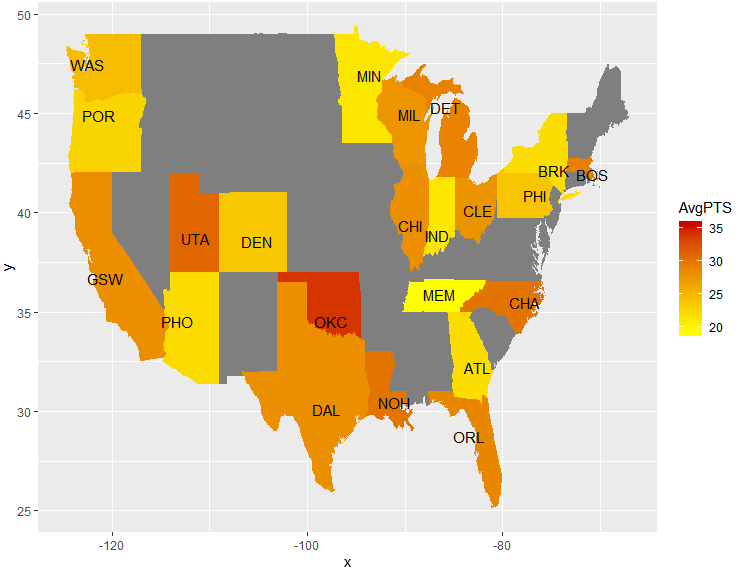区域颜色越深，表示詹姆斯在该球队所得的分数越高。
用ggplot2画美国地图是相当的方便。
实例二：新冠肺炎
方法一
数据是截至2020年2月28日00：00，各省份的累计确诊病例数
library(sp)
library(maptools);
getColor=function(mapdata,provname,provcol,othercol)
{
f=function(x,y) ifelse(x %in% y,which(y==x),0);
colIndex=sapply(mapdata$NAME,f,provname); fg=c(othercol,provcol)[colIndex+1]; return(fg); #mapdata 存放地图数据的变量x #provname 需要改变颜色的地区的名称 #provcol 对应于provname的代表颜色的向量（名称或数字） #othercol 是其它地区的颜色 } provname=c("北京市","天津市","河北省","山西省","内蒙古自治区", "辽宁省","吉林省","黑龙江省","上海市","江苏省", "浙江省","安徽省","福建省","江西省","山东省", "河南省","湖北省","湖南省","广东省", "广西壮族自治区","海南省","重庆市","四川省","贵州省", "云南省","西藏自治区","陕西省","甘肃省","青海省", "宁夏回族自治区","新疆维吾尔自治区","台湾省", "香港特别行政区"); pop=c(410,136,318,133,75,121,93,480,337,631,1205,990,296,935,756,1272,65914,1017,1348,252,168, 576,538,146,174,1,245,91,18,72,76,32,93); provcol=grey((1-pop/max(pop))) plot(x,col=getColor(x,provname,provcol,"white"),xlab="",ylab="");  颜色数据部分运行如下：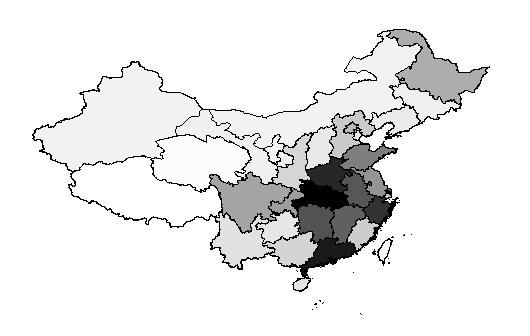方法二 将csv文件中的省份数据和程序包中所给的地图数据作矩阵合并 data<-read.csv("C:/Users/SAMSUNG/Desktop/data.csv",T) #读取人口数据 names(data) china_data <- join(china_map_data, data, type="full") #矩阵合并 names(china_data) ggplot(data,aes(x=long,y=lat,id=id,fill=number))+ #以人口数填充色彩 geom_polygon(colour="grey40")+ #省界颜色 scale_fill_gradient(low="white",high="steelblue")+#指定渐变填充色 coord_map("polyconic")+#指定投影方式为polyconic theme( #清除不需要的元素 panel.grid = element_blank(), panel.background = element_blank(), axis.text = element_blank(), axis.ticks = element_blank(), axis.title = element_blank(), legend.position = c(0.2,0.3) )  可能是因为GADM提供的id不同，矩阵合并时出现了问题，这个地方暂时还没解决，先留着。  展开全文大数据 可视化 • R软件的ggplot包升级以后有绘制地图的新功能，其图形元素主要是通过geom_map来实现。由于系统内maps包所自带的地图数据没有广州市的数据。其它图家地图数据则要从外部导入，本文则尝试从外部导入广州数据，然后用... R软件的ggplot包升级以后有绘制地图的新功能，其图形元素主要是通过geom_map来实现。由于系统内maps包所自带的地图数据没有广州市的数据。其它图家地图数据则要从外部导入，本文则尝试从外部导入广州数据，然后用ggplot2包进行绘图。 本例的目的是结合地理数据和房价数据，以说明广州市房价的行政区分布情况。基本步骤是先导入地理信息数据，将其转为ggplot2可以处理的数据框格式，将房价数据整合进来，最后绘制成图。在下图中，颜色越深代表房价越高。由于本人只在网络上下载到旧版的广州市地图，所以与现实是有些出入的。广州市行政区gis图shp格式.rar接下来展示本案例的代码 library(maptools); library(ggplot2) library(plyr) gzmap<-readShapePoly('C:/Users/Thinkpad/Desktop/广州市行政区划/guangzhou.shp') head(fortify(gzmap)) ggplot(gzmap,aes(x=long,y=lat,group=group))+geom_path() gzfj<-data.frame(行政区=c('白云区','海珠区','黄埔区','荔湾区','天河区','越秀区','花都','南沙区', '增城','从化','番禺区','萝岗区'), 房价=c(14740,17476,12387,16123,20319,26176,8541,9062,8520,8636,13838,14555)) gz.map<-fortify(gzmap,gzmap$NAME_EN)
gzdata<-data.frame(id =unique(gz.map$id),name=gzmap$NAME_EN)
gzdata1<-merge(gzfj,gzdata,by.x='行政区',by.y='name')
gz_map<-merge(gz.map,gzdata1,by.x='id',by.y='id')
gz_map<-arrange(gz_map,group,order)
ggplot(gz_map,aes(map_id = id))+
geom_map(aes(fill = 房价), map =gz.map,colour='black') +
expand_limits(x = gz.map$long, y = gz.map$lat)+theme(
panel.grid=element_blank(),
panel.background=element_blank(),
axis.text=element_blank(),
axis.title=element_blank())自动生成的颜色太暗，而且是颜色越浅代表房价越高，这些不太符合人们的思维惯性，下一步要改变颜色

改变颜色

ggplot(gz_map,aes(map_id = id))+
geom_map(aes(fill = 房价),map =gz.map,colour='black') +
expand_limits(x = gz.map$long, y = gz.map$lat)+
ggtitle("广州房价分布图")+
scale_fill_gradient2(low='#F0F5FD',mid='#85B0F2',high='#0808E7',midpoint=median(gzfj$房价))+ theme(panel.grid=element_blank(), panel.background=element_blank(), axis.text=element_blank(), axis.title=element_blank())改变颜色 ggplot(gz_map,aes(map_id = id))+ geom_map(aes(fill = 房价),map =gz.map,colour='black') + expand_limits(x = gz.map$long, y = gz.map$lat)+ ggtitle("广州房价分布图")+ scale_fill_gradient(high = "darkblue",low = "lightblue")+ theme(panel.grid=element_blank(), panel.background=element_blank(), axis.text=element_blank(), axis.title=element_blank())已经为行政区根据房价的不同涂上了不同的颜色，但是我们还想再地图上显示行政区的名称 添加文字 gzmap<-readShapePoly('C:/Users/Thinkpad/Desktop/广州市行政区划/guangzhou.shp') gz.map<-fortify(gzmap,gzmap$NAME_EN)
gzfj<-data.frame(行政区=c('白云区','海珠区','黄埔区','荔湾区','天河区','越秀区','花都','南沙区',
'增城','从化','番禺区','萝岗区'),
房价=c(14740,17476,12387,16123,20319,26176,8541,9062,8520,8636,13838,14555))
gzdata1<-merge(gzfj,gzdata,by.x='行政区',by.y='name')
str(gzdata1)

添加经度和纬度

此处可以用一个循环来表达，无奈楼主水平有限，也在学习期间，若有真正的大牛希望看到后能指点一二

gzdata1$long<-mean(gz.map$long[gz.map$id==gzdata1$id[1］)
gzdata1$long<-mean(gz.map$long[gz.map$id==gzdata1$id[2］)
gzdata1$long<-mean(gz.map$long[gz.map$id==gzdata1$id[3］)
gzdata1$long<-mean(gz.map$long[gz.map$id==gzdata1$id[4］)
gzdata1$long<-mean(gz.map$long[gz.map$id==gzdata1$id[5］)
gzdata1$long<-mean(gz.map$long[gz.map$id==gzdata1$id[6］)
gzdata1$long<-mean(gz.map$long[gz.map$id==gzdata1$id[7］)
gzdata1$long<-mean(gz.map$long[gz.map$id==gzdata1$id[8］)
gzdata1$long<-mean(gz.map$long[gz.map$id==gzdata1$id[9］)
gzdata1$long<-mean(gz.map$long[gz.map$id==gzdata1$id[10］)
gzdata1$long<-mean(gz.map$long[gz.map$id==gzdata1$id[11］)
gzdata1$long<-mean(gz.map$long[gz.map$id==gzdata1$id[12］)
gzdata1$long<-mean(gz.map$long[gz.map$id==gzdata1$id[13］)
gzdata1$long<-mean(gz.map$long[gz.map$id==gzdata1$id[14］)

gzdata1$lat<-mean(gz.map$lat[gz.map$id==gzdata1$id[1］)
gzdata1$lat<-mean(gz.map$lat[gz.map$id==gzdata1$id[2］)
gzdata1$lat<-mean(gz.map$lat[gz.map$id==gzdata1$id[3］)
gzdata1$lat<-mean(gz.map$lat[gz.map$id==gzdata1$id[4］)
gzdata1$lat<-mean(gz.map$lat[gz.map$id==gzdata1$id[5］)
gzdata1$lat<-mean(gz.map$lat[gz.map$id==gzdata1$id[6］)
gzdata1$lat<-mean(gz.map$lat[gz.map$id==gzdata1$id[7］)
gzdata1$lat<-mean(gz.map$lat[gz.map$id==gzdata1$id[8］)
gzdata1$lat<-mean(gz.map$lat[gz.map$id==gzdata1$id[9］)
gzdata1$lat<-mean(gz.map$lat[gz.map$id==gzdata1$id[10］)
gzdata1$lat<-mean(gz.map$lat[gz.map$id==gzdata1$id[11］)
gzdata1$lat<-mean(gz.map$lat[gz.map$id==gzdata1$id[12］)
gzdata1$lat<-mean(gz.map$lat[gz.map$id==gzdata1$id[13］)
gzdata1$long<-mean(gz.map$long[gz.map$id==gzdata1$id[14］)
ggplot(gz_map,aes(map_id = id))+
geom_map(aes(fill = 房价),map =gz.map,colour='black') +
expand_limits(x = gz.map$long, y = gz.map$lat)+
ggtitle("广州房价分布图")+
scale_fill_gradient2(low='#F0F5FD',mid='#85B0F2',high='#0808E7',midpoint=median(gzfj$房价))+ geom_text(aes(x =long,y = lat,label = 行政区), family = "GB1", data = gzdata1)+ theme(panel.grid=element_blank(), panel.background=element_blank(), axis.text=element_blank(), axis.title=element_blank()) 以上程序运行后就得到我们最初看到的图形了，颜色根据房价的高低来定，地图上还标注了行政区的名称。展开全文• 发一篇教大家如何用R语言画路线图的攻略~ ~\(≧▽≦)/~ 题目是这样的： 利用R地图包，提取武汉地图，并图上标注一条从华中师范大学北门到黄鹤楼的路线.  发一篇教大家如何用R语言画路线图的攻略~ ~\(≧▽≦)/~ 题目是这样的： 利用R的地图包，提取武汉地图，并在图上标注一条从华中师范大学北门到黄鹤楼的路线. 首先，读取需要用到的R包~ library(maptools) library(sp) library(ggplot2) library(mapproj)  再然后，在国家基础地理信息中心上下载地级市的shp地图包~这个网站我当时试了好多次还是登不上去，又从网上找了一个数据包~ 当然你找百度地图，谷歌地图神马的，只要能拿到市级地图都行~R语言自带的包没有精确到市级。。。 http://pan.baidu.com/s/1hs03gUg（如果分享过期了可以上网搜索BOUNT_poly.shp） 读入地级市包里的这个文件 mydat = readShapePoly("d:/rstudy/BOUNT_poly.shp") 按照99年制定的名字标准找到武汉市 tmp = iconv(mydat$NAME99, from = "GBK")
grep("武汉", tmp, value = TRUE)


mydat$ADCODE99[grep("武汉", tmp)]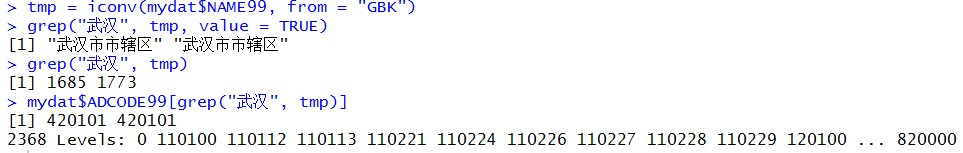接下来就是把地图数据从地图包里找出来啦~稍微改一下名称~ Wuhan = mydat[substr(as.character(mydat$ADCODE99), 1, 4) == "4201",]
mysh = fortify(Wuhan)
mysh = transform(mysh, id = iconv(id, from = 'GBK'), group = iconv(group, from = 'GBK'))
names(mysh)[1:2] = c("x","y")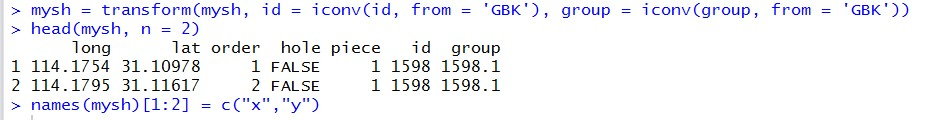为了好看，要涂上漂亮哒颜色~不过作为一个重度选择困难症，还是用随机数涂颜色啦~
（其实是因为网上的教程里有现成的随机数涂色╮(╯▽╰)╭）
先弄一串随机数把~
myepidat = data.frame(id = unique(sort(mysh$id))) myepidat$rand = runif(length(myepidat$id)) myepidat  接下来查出华师和黄鹤楼的大致经纬度，我在百度地图找的，四舍五入一下~ 然后准备好名字标签。 多个多维向量做图处理的时候记得转化成数据框哟~ E <- c(114.31,114.37) N <- c(30.55,30.52) place <- c('黄鹤楼','华师') data <- data.frame(E,place,N)  最后画出地图，涂色，标出目标点，连线。（可能是游戏打多了，用的红到绿的渐变色，地点用的是黄色，连线用蓝色~不要嘲笑我哒审美，我可是试了好多颜色哒~） ╮(╯▽╰)╭ csmap = ggplot(myepidat) + geom_map(aes(map_id = id, fill = rand), color = "white", map = mysh) + scale_fill_gradient(high = "red",low = "darkgreen") + geom_point(aes(E, N),data = data,colour="yellow") + geom_line(aes(E, N),data = data, colour = "blue",size = 1.5)+ geom_text(data = data,aes(E, N, label = place),hjust = 1.2,size = 3) + expand_limits(mysh) + coord_map() print(csmap)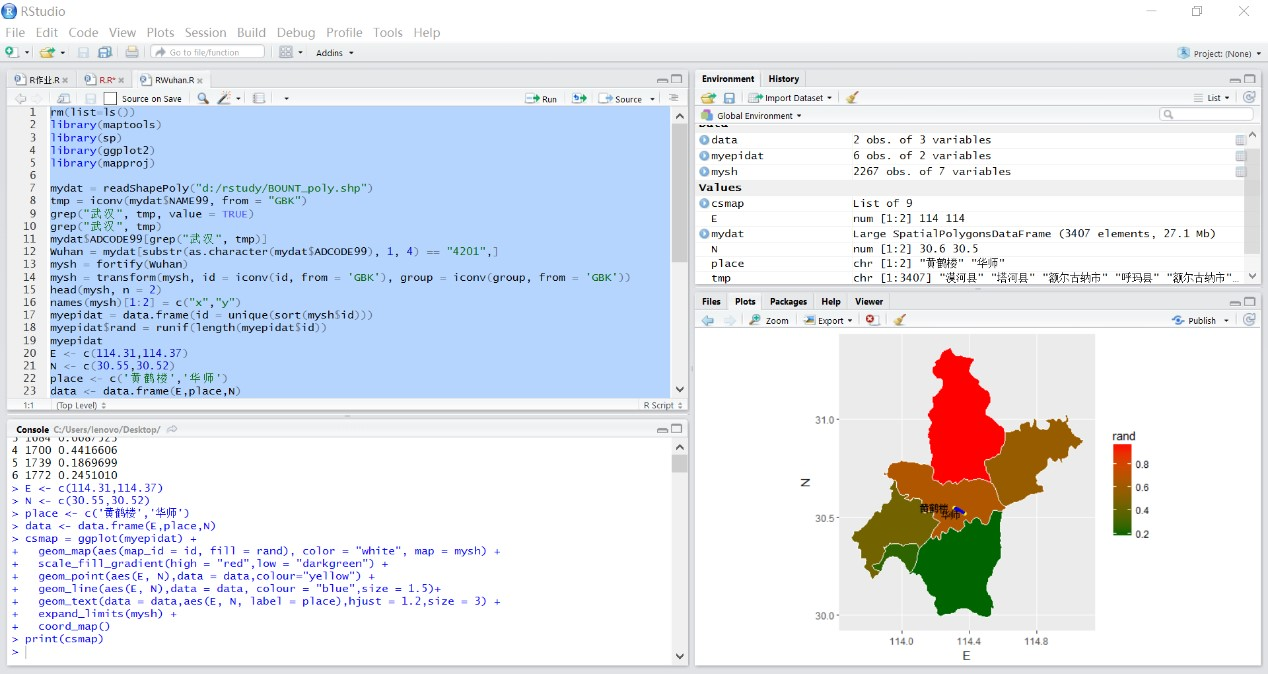全部代码： library(maptools) library(sp) library(ggplot2) library(mapproj) mydat = readShapePoly("d:/rstudy/BOUNT_poly.shp") tmp = iconv(mydat$NAME99, from = "GBK")
grep("武汉", tmp, value = TRUE)
grep("武汉", tmp)
mydat$ADCODE99[grep("武汉", tmp)] Wuhan = mydat[substr(as.character(mydat$ADCODE99), 1, 4) == "4201",]
mysh = fortify(Wuhan)
mysh = transform(mysh, id = iconv(id, from = 'GBK'), group = iconv(group, from = 'GBK'))
names(mysh)[1:2] = c("x","y")
myepidat = data.frame(id = unique(sort(mysh$id))) myepidat$rand = runif(length(myepidat$id)) myepidat E <- c(114.31,114.37) N <- c(30.55,30.52) place <- c('黄鹤楼','华师') data <- data.frame(E,place,N) csmap = ggplot(myepidat) + geom_map(aes(map_id = id, fill = rand), color = "white", map = mysh) + scale_fill_gradient(high = "red",low = "darkgreen") + geom_point(aes(E, N),data = data,colour="yellow") + geom_line(aes(E, N),data = data, colour = "blue",size = 1.5)+ geom_text(data = data,aes(E, N, label = place),hjust = 1.2,size = 3) + expand_limits(mysh) + coord_map() print(csmap)  运行结果：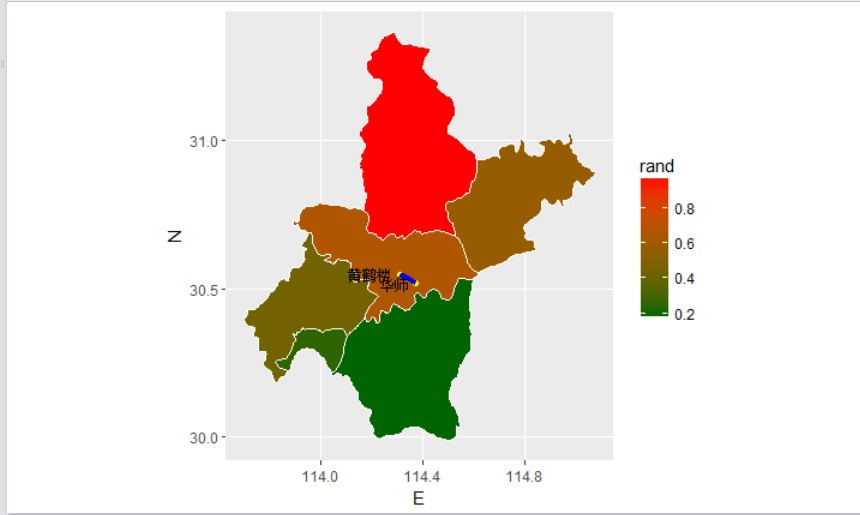参考资料：  姜晓东. R 绘制中国地图，并展示流行病学数据 https://cosx.org/2014/08/r-maps-for-china  blbailei. 如何向ggplot图中添加参考线和标准的例子http://www.dataguru.cn/thread-274407-1-1.html  展开全文• ## 利用R绘制漂亮的中国地图 万次阅读 多人点赞 2018-09-22 11:16:24 一个绘制中国地图的方法（R ggmap 说起用R绘制中国地图，很多有经验的老司机肯定会推荐ggmap，无论是里面自带的各种地区绘图、经纬度查询以及可以与ggplot无缝衔接绘制地区统计图，这都是R中其它绘制地图所难以超越的优势。 但奈何R经常无法获取ggmap里面的地图。当我想导入中国地图时，就会出现如下图所示的问题。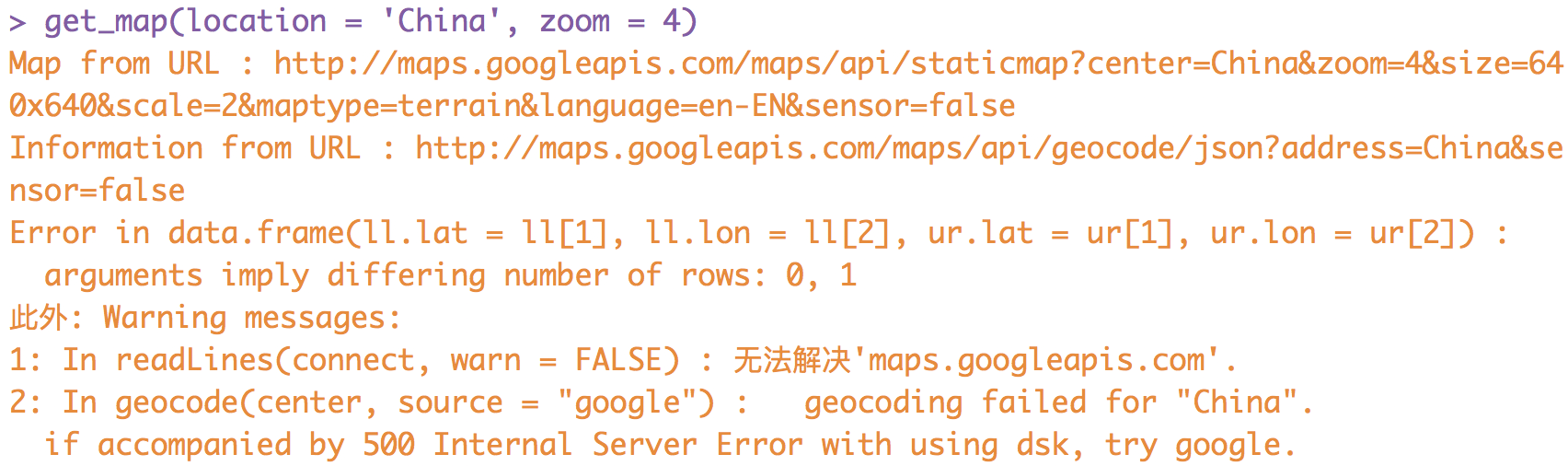由于数模所需，为了硬着头皮进行绘制，哪怕丑一些，所以探索出了下面两种绘制中国地图的方法。 maps + mapdata 这种方式绘制中国地图非常的简单，只需要一行代码（注意要先调用两个R包maps, mapdata）： map("china", col = "red4", ylim = c(18, 54), panel.first = grid())  便可以得到如下图所示的绘制结果。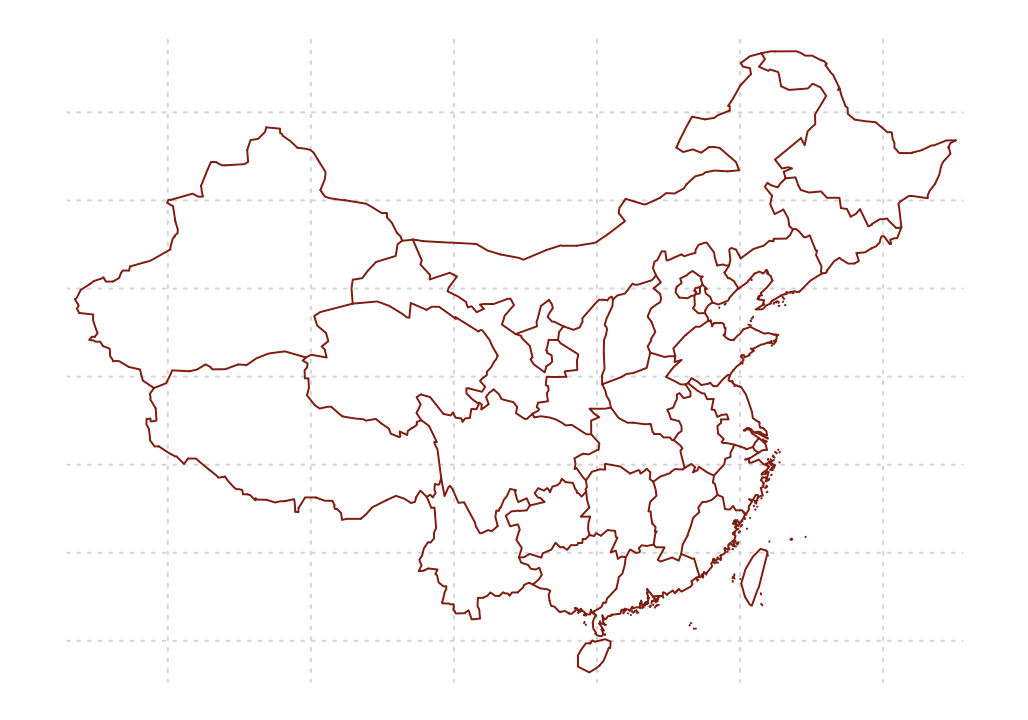但这幅图只是一幅单纯的中国地图，如果我们想要插入部分城市的人口数据以及其它信息，该如何添加呢？（数据来源：2015-2016年中国城市人口数量排名） 首先来看看我们的数据信息（代码有点丑，不要在意这些细节）： beijing = c('北京&天津', 39.90419989999999, 116.4073963, 1961.24 + 1293.82) shanghai = c('上海', 31.2303904, 121.4737021, 2301.91) zhengzhou = c('郑州', 34.7472541716, 113.6249284647, 862.65) wulumuqi = c('乌鲁木齐', 43.8266013700, 87.6168405804, 311.03) haerbin = c('哈尔滨', 45.8021755616, 126.5358247345, 1063.6) xian = c('西安', 34.3412614674, 108.9398165260, 846.78) wuhan = c('武汉', 30.5927599029, 114.3052387810, 978.54) chengdu = c('成都', 30.5702183724, 104.0647735044, 1404.76) lasa = c('拉萨', 29.6441135160, 91.1144530801, 55.94) chongqing = c('重庆', 29.5647048135, 106.5507137149, 2884.62) kunming = c('昆明', 24.8796595146, 102.8332118852, 643.2) guangshen = c('广州&深圳', 23.0206747828, 113.7517837567, 1270.08 + 1035.79) cities = c(beijing, shanghai, zhengzhou, wulumuqi, haerbin, xian, wuhan, chengdu, lasa, chongqing, kunming, guangshen) mat.cities = as.data.frame(matrix(cities, ncol = 4, byrow = T), stringsAsFactors = F) names(mat.cities) = c('names', 'lat', 'long', 'population') mat.cities$population = as.numeric(as.character(mat.cities$population)) / 100 mat.cities$lat = as.numeric(as.character(mat.cities$lat)) mat.cities$long = as.numeric(as.character(mat.cities$long))  具体操作其实也非常简单：使用for循环搭配基础的points()函数，使用如下所示的代码即可： map("china", col = "red4", ylim = c(18, 54), panel.first = grid()) for (i in 1:12) { points(mat.cities$long[i], mat.cities$lat[i], cex = 1.5, pch = 16) } title('Population')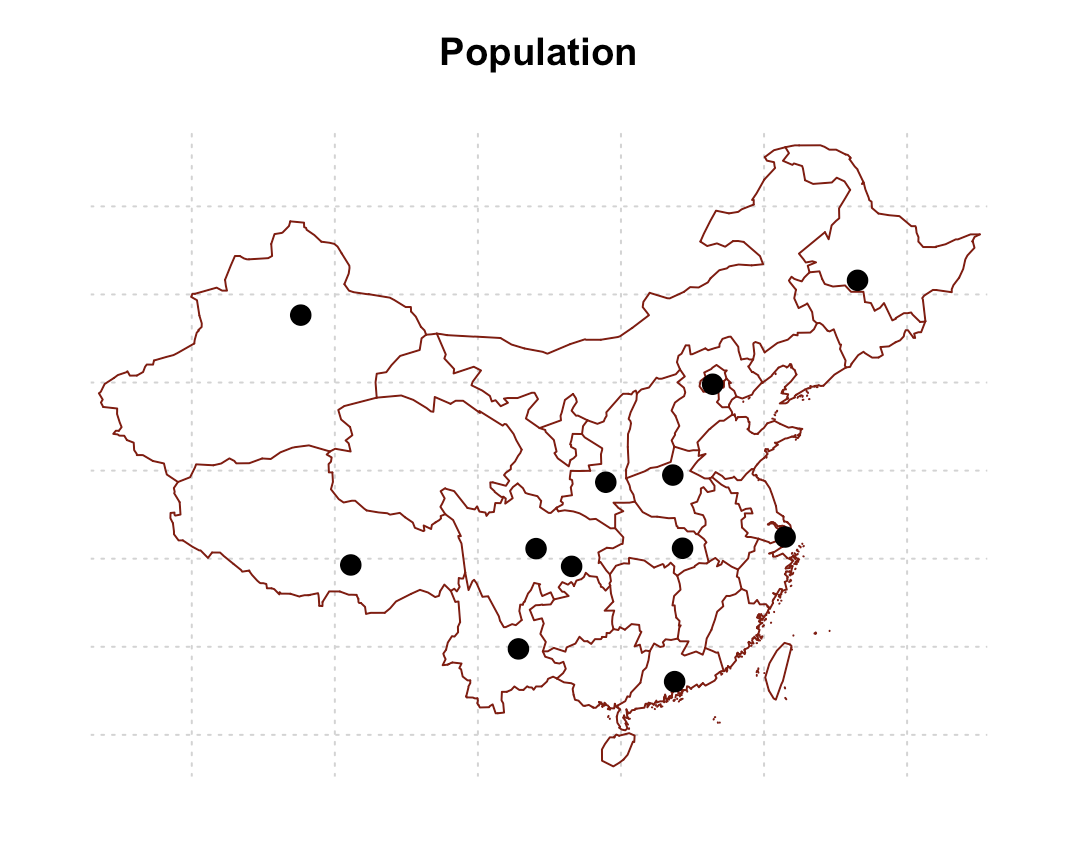这里应该还需要用其它信息来展现我们的人口数，但是由于这个绘图不是很漂亮，所以就不进行接下来的操作了。后面介绍一个更漂亮的不翻墙绘制人口地图的方法——ggplot + mapdata。 ggplot2 + mapdata 话不多说，直接用我们前面生成的数据进行绘制。这里讲一下大概的流程： 同上一种方式，利用map()函数产生中国地图的轮廓（如果能上google，使用ggmap当然更好）。 使用ggplot进行绘图: 使用geom_path()函数将我们生成的中国地图的轮廓绘制出来，这里修改颜色，选择清新自然的粉色； 用geom_point()将各个城市的位置用散点点上，同时点的大小表示人口的多少，这里用清新蓝； 用geom_text()在每个点上添加上城市的名称； 修改各个标签以及各种title的名称； 进行修改主题、Mac上显示中文字等操作。 china <- map("china", plot = F) ggplot() + geom_path(data = china, aes(long, lat, group = group), color = '#FD9FA4', show.legend = F) + geom_point(data = mat.cities, aes(x = long, y = lat, size = population), alpha = 0.8, color = '#8BB6D6') + # geom_text_repel(data = mat.cities, aes(x = long, y = lat, label = names), family = "STHeiti") + geom_text(data = mat.cities, aes(x = long, y = lat, label = names), family = "STHeiti") + labs(x = '经度', y = '纬度', title = '中国十二个地区人口地图', size = '人口(百万)') + theme_bw() + theme(panel.border = element_blank(), text = element_text(family = "STHeiti"), plot.title = element_text(hjust = 0.5))  产生出来的图像如下图所示：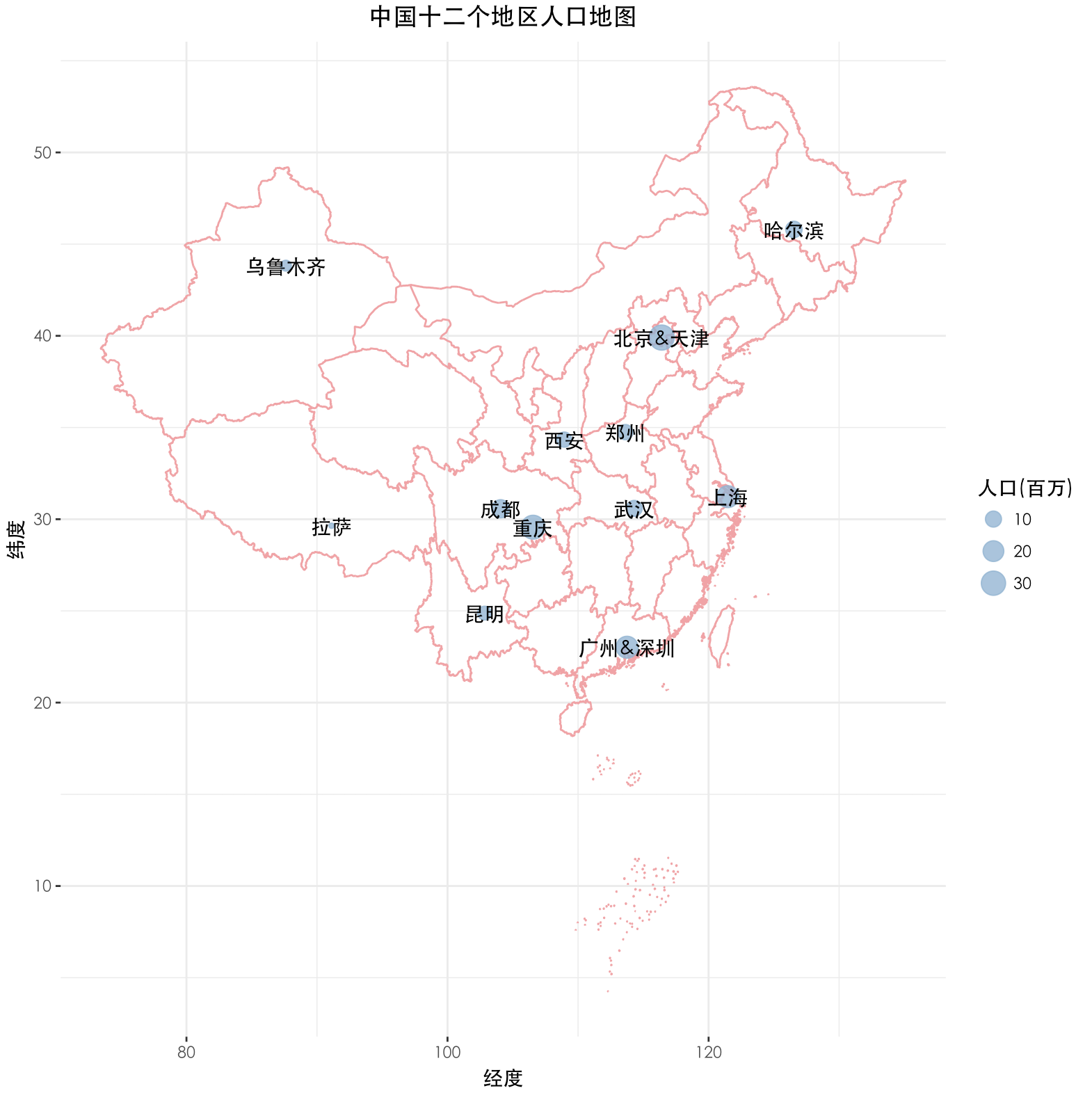这样的图已经基本算作是能看的了，但这并不是强迫症患者所最终追求的绘图。其美中不足的是每个城市的名称覆盖在我们的散点上了。 通常我们的做法非常简单，在geom_text()中设置参数，将所有的文字向上下左右选一个方向，防止覆盖。这样做当然没问题，这里介绍另一种方法，可以进行自适应调整以防覆盖。 ggrepel 我们的做法是使用ggrepel包中的geom_text_repel()函数替换掉我们原本的geom_text()，细心的同学已经发现，这就是我们上面代码注释掉的部分。这时只需将其封印解除，同时封印住geom_text()这个小顽皮，我们的绘图就大功告成了！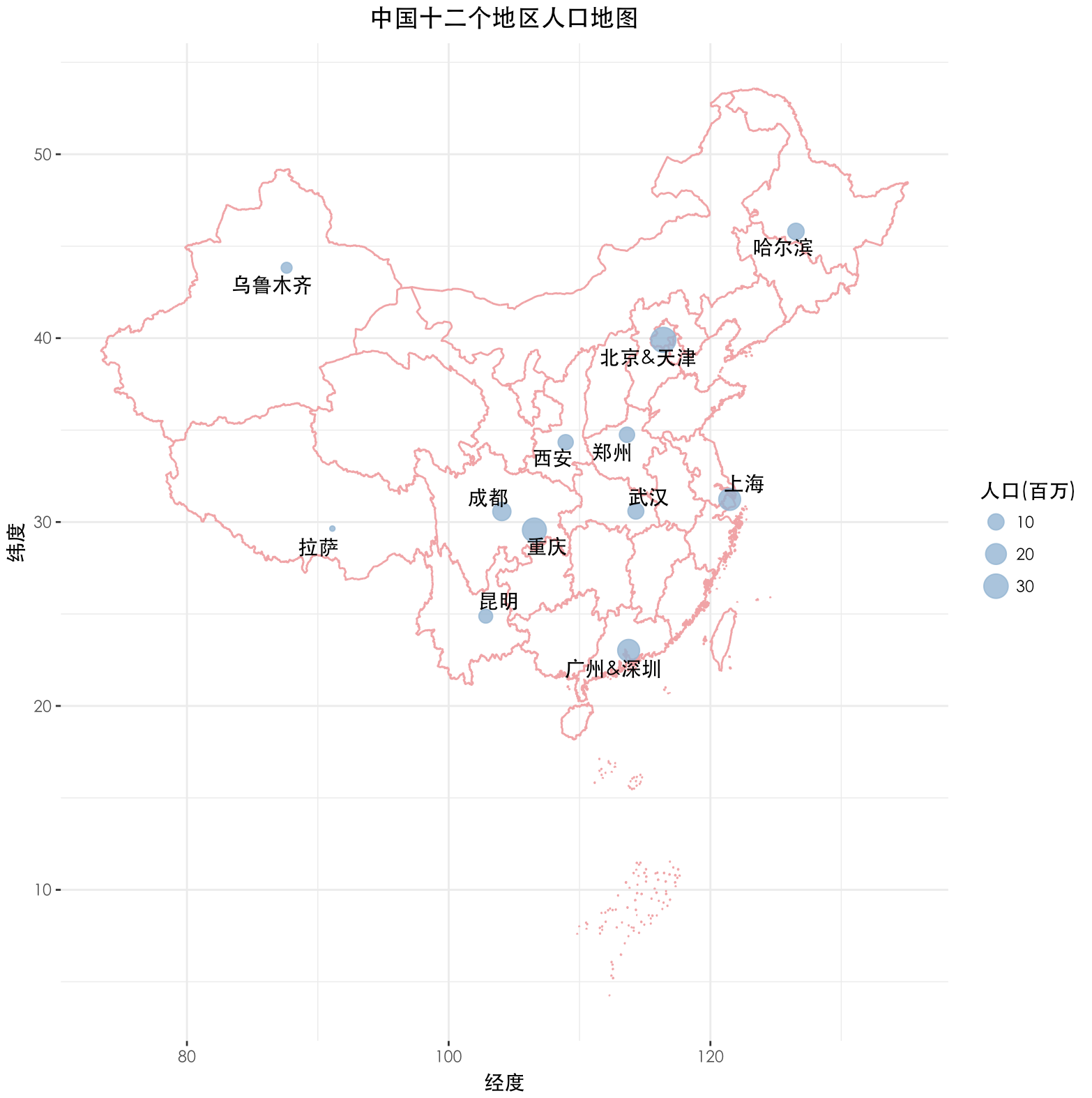展开全文• 最近更是新出了几款插件，能在线预览文档，能做GIS数据地图，还能集成R语言！ 这还是我认识的报表工具？ 没错，就是FineReport ！ 一起来看看这几个锦上添花的功能吧！ 1、集成R语言 在数据分析、数据统计和...大数据 商业智能 • 本教程，您将学习如何将中等大小的数据集（如游戏元数据）转换为有用的格式，以便使用R进行进一步分析。 您将了解整洁数据集遵循的关键原则，为什么跟踪它们有用，以及如何清理您给出的数据。整理也是了解新... • 数据分析的流程主要包括以下几个4大步骤，而熟练掌握以下每个步骤的常用包，就能提高数据科学的效率与质量。 1.数据读取 2.数据清洗 3.数据可视化 4.数据挖掘 细分为以下流程： 1.1 数据导入 1.2 数据整理... • ## R语言-地图绘制的思路 千次阅读 多人点赞 2020-02-26 16:49:47 R中的画地图的方法不外乎两种，一种是利用包里GIS方面的数据在R中直接画出来，另一种是从其他地方拿到数据在R中通过某些包解析后再展现成。这两种方法只是数据来源不同，具体的画图以及美化方法无异。 第一种，... • R语言中ggplot2包提供绘制地图、散点图的方法，是实现矢量地图上绘制分级设色散点图核心包 绘制多边形geom_polygon（data,aes,fill, colour） 绘制点 geom_point ..... 0、需要用到的包 library(maptools) # ... • 做Javascript相关地图开发的码农，特别关心可视化和开源的同学，都听说过Leaflet这样一个神包（神马，你没有听说过……好吧，当我没说，你自己先搜索一下……） 用官方（自吹自擂）的话来说，Leaflet包是号称最受...html5 可视化 leaflet • ## R语言数据挖掘 千次阅读 2017-05-02 11:42:00 R语言数据挖掘 Learning Data Mining with R ［哈萨克斯坦］贝特·麦克哈贝尔（Bater Makhabel） 著 李洪成 许金炜 段力辉 译 图书版编目（CIP）数据 R语言数据挖掘 / （哈）贝特·麦克哈贝尔（Bater ... • 使用ggplot2在地图上绘制热力图 ######*****绘制热力图代码*****####### interval <- seq(0, 150000, 25000)[-2] #设置价格区间 newpri <- cut(data1$price,...
• 个人博客: https://ytlogos.github.io/公众号：生信大讲堂往期回顾R语言可视化学习笔记之相关矩阵可视化包ggcorrplotR语言学习笔记之相关性矩阵分析及其可视化ggplot2学习笔记系列之利用ggplot2绘制误差棒及显著性...
• 栅格数据是当前GIS领域里面最庞大的一类数据（天上N颗卫星24小时无死角的对地球君进行直播……每天生成遥感数据都以PB为单位），leaflet作为地图可视化模块，自然也提供了加载栅格数据的方法。   方法如下： ...leaflet 可视化
• 一、数据科学工作流程1.1 数据导入 1.2 数据整理 1.3 反复理解数据 1.4 数据可视化 1.5 数据转换 1.6 统计建模 1.7 作出推断（比如预测） 1.8 沟通交流 ...在R和python上都可使用 readr：实现
• 本文分享文字识别技术高德地图数据生产的演进与实践，介绍了文字识别自研算法的主要发展历程和框架，以及未来的发展和挑战。一 背景作为一个DAU过亿的国民级软件，高德地图每天为用户提供海量的文字识别
• 导读：丰富准确的地图数据大大提升了我们使用高德地图出行的体验。相比于传统的地图数据采集和制作，高德地图大量采用了图像识别技术来进行数据的自动化生产，而其中场景文字识别技术占据了重要位置...算法 大数据 python 计算机视觉
• R地图数据 首先，从这里下载中国地图的GIS数据，这是一个压缩包，完全解压后包含三个文件（bou2_4p.dbf、bou2_4p.shp和bou2_4p.shx），将这三个文件解压到同一个目录下。 用R绘制地图比较简单。比如画一下...
• R语言数据分析、展现与实例（02）数据输入> mydata (age=numeric(0),gender=character(0),weight=numeric(0)) #创建空数据框 > mydata (mydata) #打开编辑框进行编辑，并将结果赋值给原数据框　 输入数据，直接退出...
• 内容是使用R语言进行带南海九段线分位数地图可视化。虾神的原博文地址如下（Python版）。 Python实现带南海九段线分位数地图完整可视化版本（附代码及数据） 1999-2017年中国各省旅游外汇收入分析及可视化（附代码及...
• 1975年以后至今，计算机作为先驱，数据可视化得以蓬勃发展，成为数据挖掘和数据分析行业与受众沟通的基本形式，数据可视化成了数据与受众沟通的语言，使数据发出决策的指令。本章的目的是领悟数据可视化的科学性、...
• 四、标记点标记在地图符号化的时候，是最常用的功能之一，一般来说，带有经纬度坐标的点信息，在地图上标志的位置，都会通过一个标记物来实现，很多时候把这个东东称之为“图钉”是非常形象的。标记的方法名和语法......